# Galilean Transformation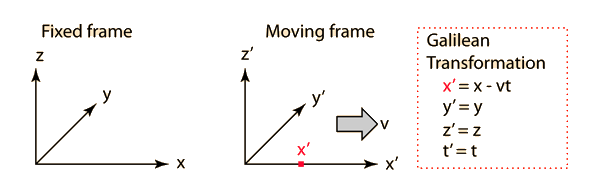### Show lorentz transformation

Index

Relativity concepts

 HyperPhysics***** Relativity R Nave
Go Back

# Lorentz Transformation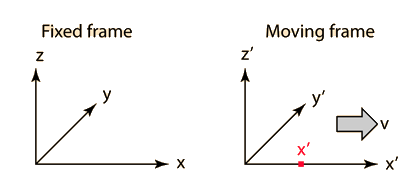The primed frame moves with velocity v in the x direction with respect to the fixed reference frame. The reference frames coincide at t=t'=0. The point x' is moving with the primed frame.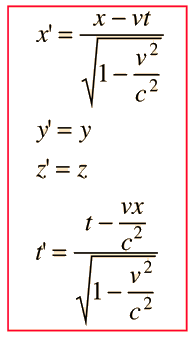The reverse transformation is: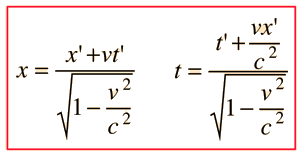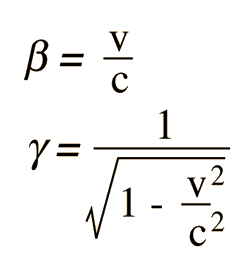Much of the literature of relativity uses the symbols β and γ as defined here to simplify the writing of relativistic relationships.

 Evaluation of symbols

### Show Galilean transformation

 Length contraction Time dilation
 Lorentz transformation in 4-vector form
Index

Relativity concepts

 HyperPhysics***** Relativity R Nave
Go Back

# Speed of Light

Experimental measurements of the speed of light have been refined in progressively more accurate experiments since the seventeenth century. Recent experiments give a speed of

c = 299,792,458 ± 1.2 m/s

but the uncertainties in this value are chiefly those of comparisons to previous standards for the length of the meter. Therefore the above speed of light has been adopted as a standard value and the length of the meter is redefined to be consistent with this value.

c ≡ 299,792,458 m/s

The speed of light in a medium is related to the electric and magnetic properties of the medium, and the speed of light in vacuum can be expressed as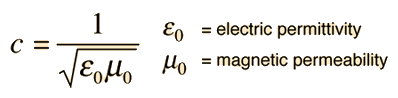In vacuum, all electromagnetic waves travel at c, the speed of light.

 c as speed limit of the universe
 Some history
Index

Relativity concepts

 HyperPhysics***** Relativity R Nave
Go Back

# Relativity Factor

Certain algebraic expressions appear so often in relativity that they are given separate symbols:Much of the literature of relativity uses the symbols β and γ as defined here to simplify the writing of relativistic relationships.

For v = c

β = and γ = .

The relativity factor shows up in:
 Length contraction: L = L0/γ = L0 Time dilation T = γT0 = T0 Relativistic mass m = γm0 = m0

For relatively low velocities where the relativity factor gamma is close to one, the binomial expansion can be used to evaluate the small relativistic corrections.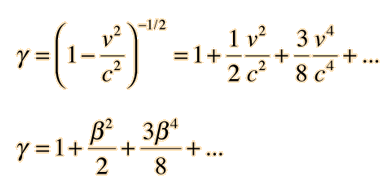Index

Relativity concepts

 HyperPhysics***** Relativity R Nave
Go Back

# c as Speed Limit

The speed of light c is said to be the speed limit of the universe because nothing can be accelerated to the speed of light with respect to you. A common way of describing this situation is to say that as an object approaches the speed of light, its mass increases and more force must be exerted to produce a given acceleration. There are difficulties with the "changing mass" perspective, and it is generally preferrable to say that the relativistic momentum and relativistic energy approach infinity at the speed of light. Since the net applied force is equal to the rate of change of momentum and the work done is equal to the change in energy, it would take an infinite time and an infinite amount of work to accelerate an object to the speed of light. (Sorry, Captain Kirk. We can't give you warp speed!)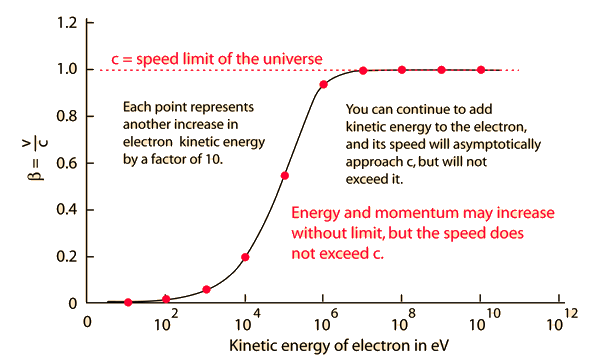A common resistance to the speed limit is to suggest that you just accelerate two different objects to more than half of the speed of light and point them toward each other, giving a relative speed greater than c. But that doesn't work! Time and space are interwoven in such a way that no one observer ever sees another object moving toward them at greater than c. The Einstein velocity addition deals with the transformation of velocities, always yielding a relative velocity less than c. It doesn't agree with your common sense, but it appears to be the way the universe works.

Index

Relativity concepts

 HyperPhysics***** Relativity R Nave
Go Back